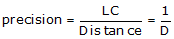## 2. Closure

On a closed loop traverse, the sum of the Lats should equal zero as should the sum of the Deps.

Closure condition:Equations D-3 and D-4Figure D-7
Perfect Closed Loop

Most traverses won’t close perfectly due to the presence of errors. If the field crew followed careful procedures and compensated distance measurements for atmospheric conditions, only random errors prevent achieving perfect closure:event achieving perfect closure:

Actual closure:Equations D-5 and D-6Figure D-8
Cumulative Random ErrorsFigure D-9 Linear Closure

The Linear Closure, LC, is the total misclosure distance. It is computed from the Lat and Dep errors:Equation D-7

Traverse precision is a function of the linear closure and the total survey distance. The precision is stated as a relative value, usually as 1 unit of error per D units measured:Equation D-8

What is allowable minimum precision? It depends on the survey purpose. Traditional first order surveys have a required precision of 1/100,000; in Wisconsin a property survey must be at least 1/3000. In order to achieve a formal minimum precision, specific equipment and field procedures must be used which match that precision requirement. Achieving a minimum precision should be by design not by accident.